Spatial domain and frequency domain filters are commonly classified into four types of filters — low-pass, high-pass, band-reject and band-pass filters. In this article I have notes, code examples and image output for each one of them.

## Types of filters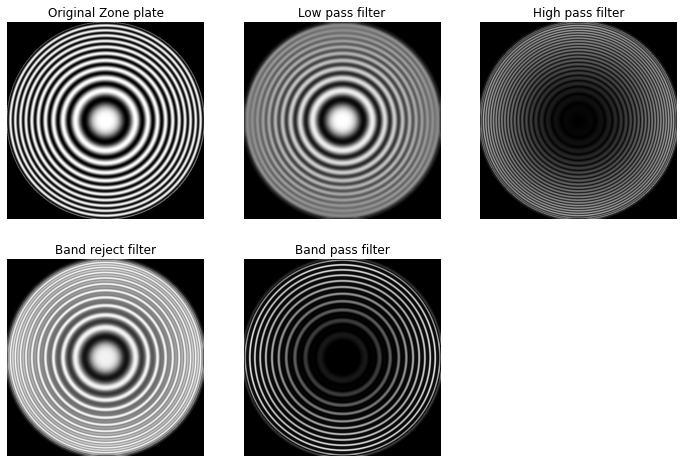• Lowpass filters: Allow passing only low frequency details, attenuates the high frequency details. Example: Smoothening filters.
• Highpass filters: Allows passing only high frequency details, attenuates the low frequency details. Example: Sharpening mask filters.
• Bandreject filters: Attenuate signal in range of a certain frequency. Allows frequency below a certain threshold and above another threshold to pass.
• Bandpass filters: Only allows signals within a certain band to pass, attenuates the frequencies below a threshold and above another threshold to pass.

### Spatial kernels in terms of low-pass kernel — `lp(x, y)`

Kernels Equation
Lowpass kernel `lp(x, y)`
Highpass kernel `hp(x, y) = δ(x, y) - lp(x, y)`
Bandreject kernel `br(x, y) = lp1(x, y) + hp2(x, y)`
`br(x, y) = lp1(x, y) + (δ(x, y) - lp2(x, y))`
Bandpass kernel `bp(x, y) = δ(x, y) - br(x, y)`

`δ(x, y)` is a unit impulse kernel

## Processing images with these filters using Zone plate

Zone plate is used for testing the characteristics of the filters. There are various versions of zone plates the one I’d be using can be generated with:

``````f(x, y) = 0.5 * (1 + cos(x^2 + y^2))
``````

Where x, y belong to `[-8.2, 8.2]` increasing in steps of `0.0275` to form a 597x597 image.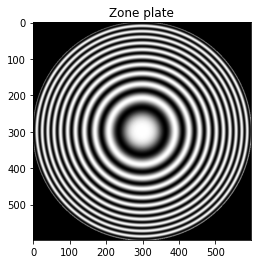``````def zone(x, y):
return 0.5 * (1 + math.cos(x * x + y * y))

SIZE = 597
image = np.zeros((SIZE, SIZE))

start = -8.2
end = 8.2
step = 0.0275

def dist_center(y, x):
global SIZE
center = SIZE / 2
return math.sqrt( (x - center)**2 + (y - center)**2)

for y in range(0, SIZE):
for x in range(0, SIZE):
if dist_center(y, x) > 300:
continue
y_val = start + y * step
x_val = start + x * step
image[y, x] = zone(x_val, y_val)
``````

## Lowpass filter

``````kernel_size = 15

lowpass_kernel_gaussian = gkern(kernel_size)
lowpass_kernel_gaussian = lowpass_kernel_gaussian / lowpass_kernel_gaussian.sum()

lowpass_kernel_box = np.ones((kernel_size, kernel_size))
lowpass_kernel_box = lowpass_kernel_box / (kernel_size * kernel_size)

lowpass_image_gaussian = cv2.filter2D(image, -1, lowpass_kernel_gaussian)
lowpass_image_box = cv2.filter2D(image, -1, lowpass_kernel_box)
``````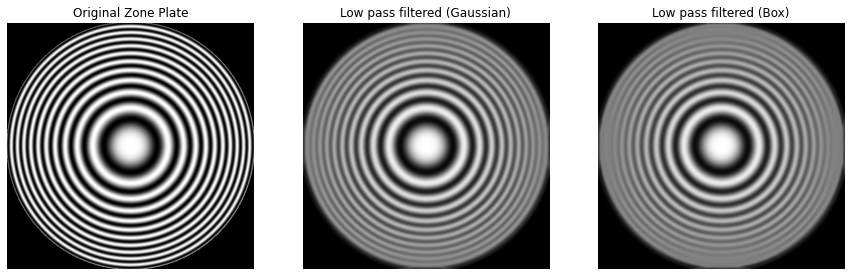## Highpass filter

In spatial domain, a Highpass filtered image can be obtained by subtracting a Lowpass filtered image from the image itself (like unsharp mask).

``````highpass_image_gaussian = image - lowpass_image_gaussian
highpass_image_gaussian = np.absolute(highpass_image_gaussian)

highpass_image_box = image - lowpass_image_box
highpass_image_box = np.absolute(highpass_image_box)
``````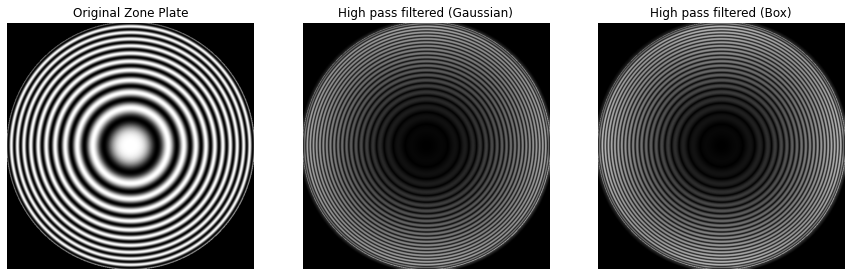## Bandreject filter

Similarly, a Bandreject filtered image can be obtained by adding a Lowpass filtered with a Highpass filtered image (at different threshold).

``````bandreject_image = lowpass_image_gaussian + highpass_image_box
``````## Bandpass filter

And Bandpass filtered image can be obtained by subtracting the Bandreject filtered image from the image itself.

``````bandpass_image = image - bandreject_image
bandpass_image = np.absolute(bandpass_image)
``````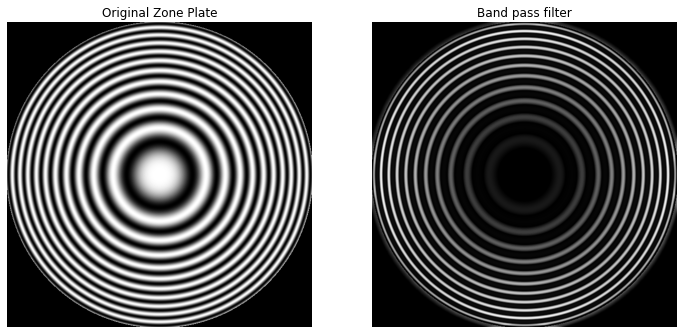## Credits

A lot of this is derived from the book Digital Image Processing — by Rafael C. Gonzalez & Richard E. Woods and can be used as quick refresher. I’ll only be listing some key pointers, sample code and the result of running them.

Disclaimer: While I do recommend this book (I use it) for learning digital image processing, if you buy this book via this link — I’ll be paid a certain small percentage in commission.